# Creating plots in R using ggplot2 - part 8: density plots

written in

This is the eighth tutorial in a series on using ggplot2 I am creating with Mauricio Vargas Sepúlveda. In this tutorial we will demonstrate some of the many options the ggplot2 package has for creating and customising density plots. We will use R’s airquality dataset in the datasets package.

Mauricio and I have also published these graphing posts as a book on Leanpub. We tend to put any changes or updates to the code in the book before these blog posts, so please check it out if you have any issues with the code examples in this post; otherwise feel free to contact us with any questions!

The first thing to do is load in the data, as below:

rm(list = ls())
library(datasets)
library(ggplot2)

data(airquality)


In this tutorial, we will work towards creating the density plot below. We will take you from a basic density plot and explain all the customisations we add to the code step-by-step.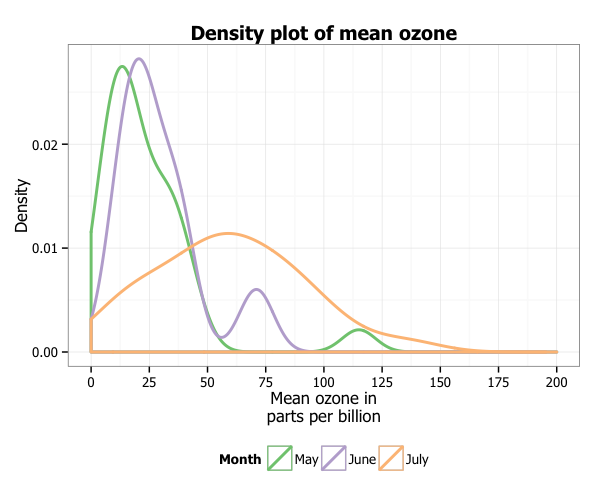### Basic density plot

In order to initialise a plot we tell ggplot that airquality is our data, and specify that our x axis plots the Ozone variable. We then instruct ggplot to render this as a density plot by adding the geom_density() option.

p8 <- ggplot(airquality, aes(x = Ozone)) +
geom_density()
p8### Customising axis labels

In order to change the axis labels, we have a couple of options. In this case, we have used the scale_x_continuous and scale_y_continuous options, as these have further customisation options for the axes we will use below. In each, we add the desired name to the name argument as a string.

p8 <- p8 + scale_x_continuous(name = "Mean ozone in parts per billion") +
scale_y_continuous(name = "Density")
p8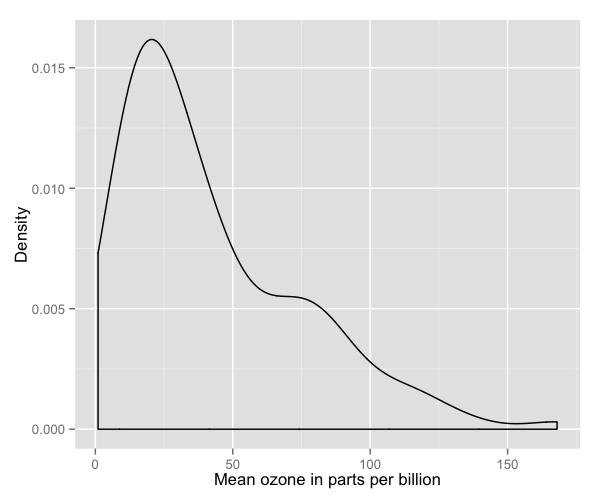ggplot also allows for the use of multiline names (in both axes and titles). Here, we’ve changed the x-axis label so that it goes over two lines using the \n character to break the line.

p8 <- p8 + scale_x_continuous(name = "Mean ozone in\nparts per billion")
p8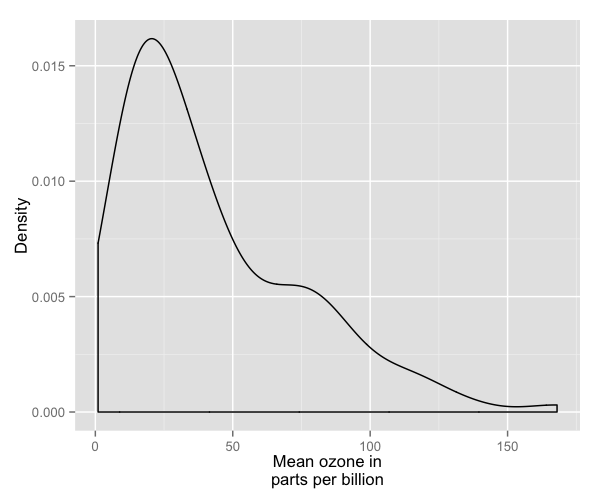### Changing axis ticks

The next thing we will change is the axis ticks. Let’s make the x-axis ticks appear at every 25 units rather than 50 using the breaks = seq(0, 200, 25) argument in scale_x_continuous. (The seq function is a base R function that indicates the start and endpoints and the units to increment by respectively. See help(seq) for more information.) We ensure that the x-axis begins and ends where we want by also adding the argument limits = c(0, 200) to scale_x_continuous.

p8 <- p8 + scale_x_continuous(name = "Mean ozone in\nparts per billion",
breaks = seq(0, 200, 25),
limits=c(0, 200))
p8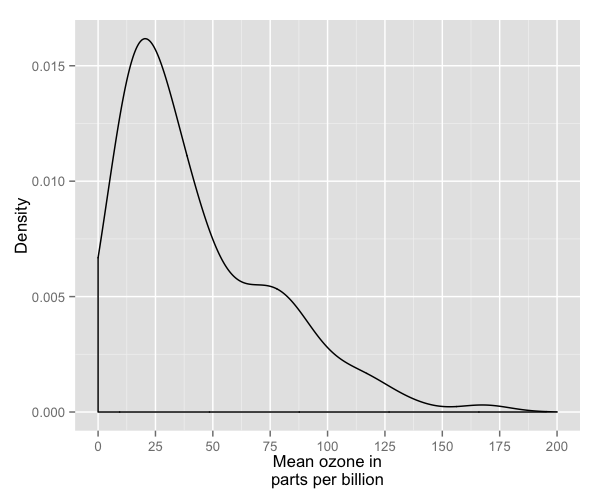### Adding a title

To add a title, we include the option ggtitle and include the name of the graph as a string argument.

p8 <- p8 + ggtitle("Density plot of mean ozone")
p8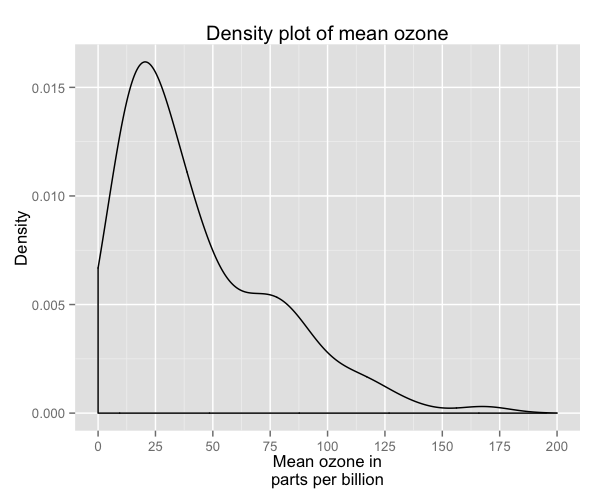### Changing the colour of the curves

To change the line and fill colours of the density plot, we add a valid colour to the colour and fill arguments in geom_density() (note that I assigned these colours to variables outside of the plot to make it easier to change them). A list of valid colours is here.

fill <- "gold1"
line <- "goldenrod2"

p8 <- ggplot(airquality, aes(x = Ozone)) +
geom_density(fill = fill, colour = line) +
scale_x_continuous(name = "Mean ozone in\nparts per billion",
breaks = seq(0, 200, 25),
limits=c(0, 200)) +
scale_y_continuous(name = "Density") +
ggtitle("Density plot of mean ozone")
p8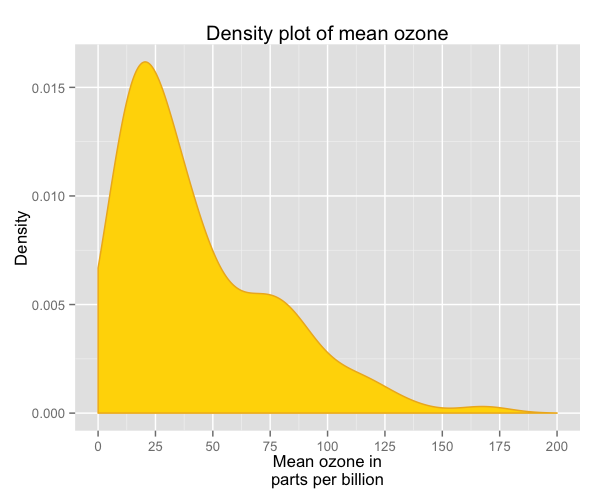If you want to go beyond the options in the list above, you can also specify exact HEX colours by including them as a string preceded by a hash, e.g., “#FFFFFF”. Below, we have called two shades of blue for the fill and lines using their HEX codes.

fill <- "#4271AE"
line <- "#1F3552"

p8 <- ggplot(airquality, aes(x = Ozone)) +
geom_density(fill = fill, colour = line) +
scale_x_continuous(name = "Mean ozone in\nparts per billion",
breaks = seq(0, 200, 25),
limits=c(0, 200)) +
scale_y_continuous(name = "Density") +
ggtitle("Density plot of mean ozone")
p8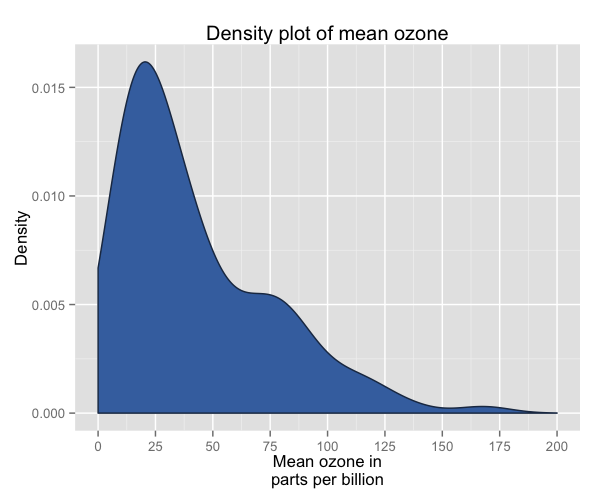You can also specify the degree of transparency in the density fill area using the argument alpha in geom_density. This ranges from 0 to 1.

p8 <- ggplot(airquality, aes(x = Ozone)) +
geom_density(fill = fill, colour = line,
alpha = 0.6) +
scale_x_continuous(name = "Mean ozone in\nparts per billion",
breaks = seq(0, 200, 25),
limits=c(0, 200)) +
scale_y_continuous(name = "Density") +
ggtitle("Density plot of mean ozone")
p8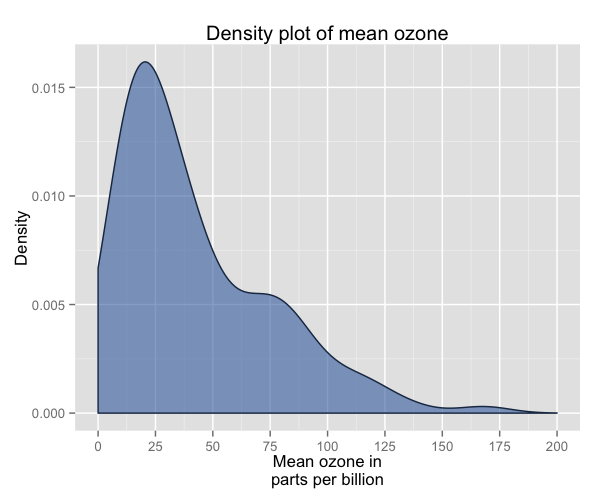### Using the white theme

As explained in the previous posts, we can also change the overall look of the plot using themes. We’ll start using a simple theme customisation by adding theme_bw(). As you can see, we can further tweak the graph using the theme option, which we’ve used so far to change the legend.

p8 <- p8 + theme_bw()
p8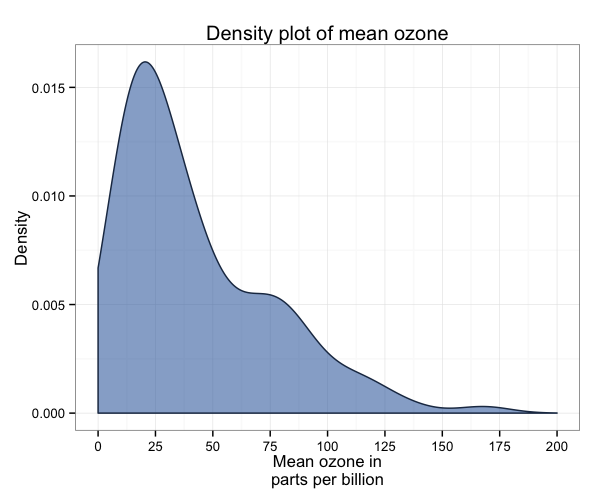### Creating an XKCD style chart

Of course, you may want to create your own themes as well. ggplot2 allows for a very high degree of customisation, including allowing you to use imported fonts. Below is an example of a theme Mauricio was able to create which mimics the visual style of XKCD. In order to create this chart, you first need to import the XKCD font, install it on your machine and load it into R using the extrafont package. These instructions are taken from here:

library(extrafont)

dest="xkcd.ttf", mode="wb")
system("mkdir ~/.fonts")
system("cp xkcd.ttf  ~/.fonts")
font_import(paths = "~/.fonts", pattern="[X/x]kcd")
fonts()


You can then create your graph:

p8 <- ggplot(airquality, aes(x = Ozone)) +
geom_density(colour = "black", fill = "#56B4E9") +
scale_x_continuous(name = "Mean ozone in\nparts per billion",
breaks = seq(0, 200, 25),
limits=c(0, 200)) +
scale_y_continuous(name = "Density") +
ggtitle("Density plot of mean ozone") +
theme(axis.line = element_line(size=1, colour = "black"),
panel.grid.major = element_blank(),
panel.grid.minor = element_blank(),
panel.border = element_blank(),
panel.background = element_blank(),
plot.title=element_text(size = 20, family="xkcd-Regular"),
text=element_text(size = 16, family="xkcd-Regular"),
axis.text.x=element_text(colour="black", size = 12),
axis.text.y=element_text(colour="black", size = 12))
p8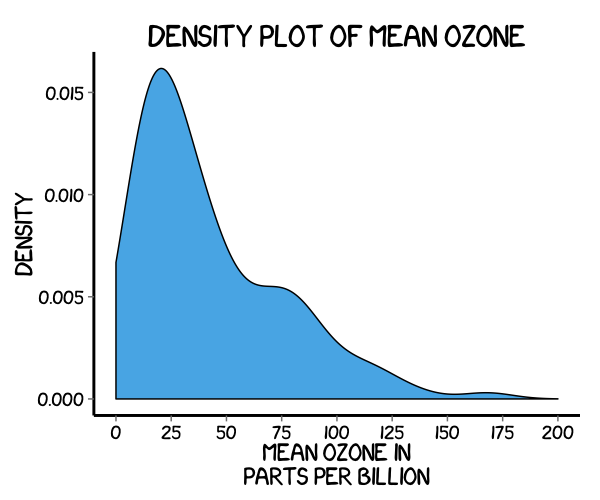### Using ‘The Economist’ theme

There are a wider range of pre-built themes available as part of the ggthemes package (more information on these here). Below we’ve applied theme_economist(), which approximates graphs in the Economist magazine.

library(ggthemes)
library(grid)

fill <- "#4271AE"
line <- "#1F3552"

p8 <- ggplot(airquality, aes(x = Ozone)) +
geom_density(fill = fill, colour = line) +
scale_x_continuous(name = "Mean ozone in\nparts per billion",
breaks = seq(0, 200, 25),
limits=c(0, 200)) +
scale_y_continuous(name = "Density") +
ggtitle("Density plot of mean ozone") +
theme_economist() +
theme(legend.position = "bottom", legend.direction = "horizontal",
legend.box = "horizontal",
legend.key.size = unit(1, "cm"),
plot.title = element_text(family="Tahoma"),
text = element_text(family = "Tahoma"),
axis.title = element_text(size = 12),
legend.text = element_text(size = 9),
legend.title=element_text(face = "bold", size = 9))
p8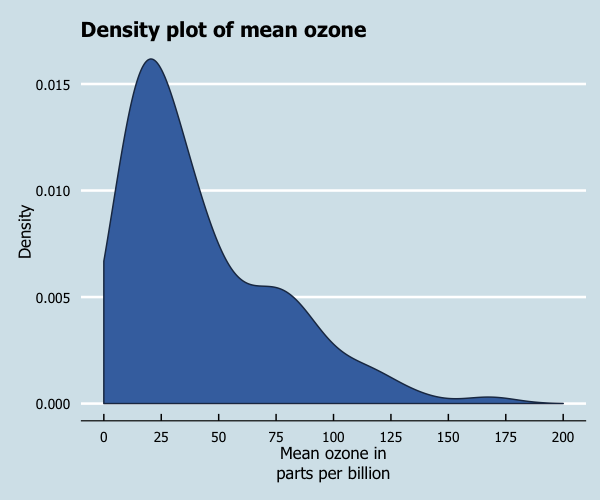### Creating your own theme

As before, you can modify your plots a lot as ggplot2 allows many customisations. Here is a custom plot where we have modified the axes, background and font.

library(grid)

fill <- "#4271AE"
lines <- "#1F3552"

p8 <- ggplot(airquality, aes(x = Ozone)) +
geom_density(colour = lines, fill = fill,
size = 1) +
scale_x_continuous(name = "Mean ozone in\nparts per billion",
breaks = seq(0, 200, 25),
limits=c(0, 200)) +
scale_y_continuous(name = "Density") +
ggtitle("Density plot of mean ozone") +
theme_bw() +
theme(axis.line = element_line(size=1, colour = "black"),
panel.grid.major = element_line(colour = "#d3d3d3"),
panel.grid.minor = element_blank(),
panel.border = element_blank(), panel.background = element_blank(),
plot.title = element_text(size = 14, family = "Tahoma", face = "bold"),
text=element_text(family="Tahoma"),
axis.text.x=element_text(colour="black", size = 9),
axis.text.y=element_text(colour="black", size = 9))
p8Let’s say that we want to add a cutoff value to the chart (75 parts of ozone per billion). We add the geom_vline option to the chart, and specify where it goes on the x-axis using the xintercept argument. We can customise how it looks using the colour and linetype arguments in geom_vline. (In the the same way, horizontal lines can be added using the geom_hline.)

fill <- "#4271AE"
line <- "#1F3552"

p8 <- p8 + geom_vline(xintercept = 75, size = 1, colour = "#FF3721",
linetype = "dashed")
p8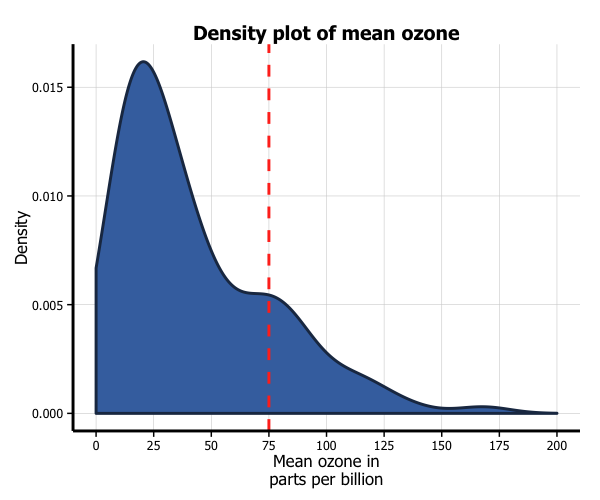### Multiple densities

You can also easily create multiple density plots by the levels of another variable. There are two options, in separate (panel) plots, or in the same plot. There are also a couple of variations on these we’ll discuss below.

We first need to do a little data wrangling. In order to make the graphs a bit clearer, we’ve kept only months “5” (May), “6” (June) and “7” (July) in a new dataset airquality_trimmed. We also need to convert this variable into either a character or factor variable. We have created a new factor variable Month.f.

In order to produce a panel plot by month, we add the facet_grid(. ~ Month.f) option to the plot. Note that we’ve also changed the scale of the x-axis to make it fit a little more neatly in the panel format.

airquality_trimmed <- airquality[which(airquality$Month == 5 | airquality$Month == 6 |
airquality$Month == 7), ] airquality_trimmed$Month.f <- factor(airquality_trimmed\$Month,
labels = c("May", "June", "July"))

p8 <- ggplot(airquality_trimmed, aes(x = Ozone)) +
geom_density(fill = fill, colour = line,
alpha = 0.6) +
scale_x_continuous(name = "Mean ozone in\nparts per billion",
breaks = seq(0, 200, 50),
limits=c(0, 200)) +
scale_y_continuous(name = "Density") +
ggtitle("Density plot of mean ozone") +
theme_bw() +
theme(plot.title = element_text(size = 14, family = "Tahoma", face = "bold"),
text = element_text(size = 12, family = "Tahoma")) +
facet_grid(. ~ Month.f)
p8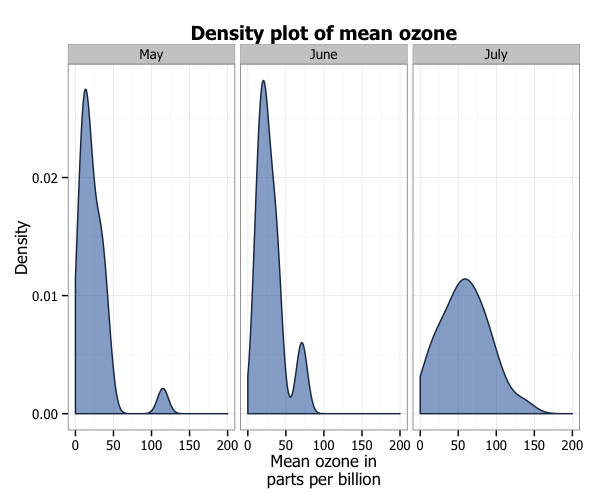An alternative to a panel plot is the volcano plot. This plot swaps the axes (so the variable of interest is on the y-axis and the density is on the x-axis), and reflects the density. In order to create this plot, we replace geom_density with stat_density, and include the arguments aes(ymax = ..density.., ymin = -..density..) and geom = "ribbon" to create a density plot, the usual fill, colour and alpha arguments, and position = "identity". We also need to add a coord_flip() option to the plot.

p8 <- ggplot(airquality_trimmed, aes(x = Ozone)) +
stat_density(aes(ymax = ..density..,  ymin = -..density..),
geom = "ribbon",
fill = fill, colour = line, alpha = 0.6,
position = "identity") +
scale_x_continuous(name = "Mean ozone in\nparts per billion",
breaks = seq(0, 200, 25),
limits=c(0, 200)) +
scale_y_continuous(name = "Density",
breaks = seq(-0.03, 0.03, 0.03)) +
ggtitle("Density plot of mean ozone") +
theme_bw() +
theme(plot.title = element_text(size = 14, family = "Tahoma", face = "bold"),
text = element_text(size = 12, family = "Tahoma")) +
facet_grid(. ~ Month.f) +
coord_flip()
p8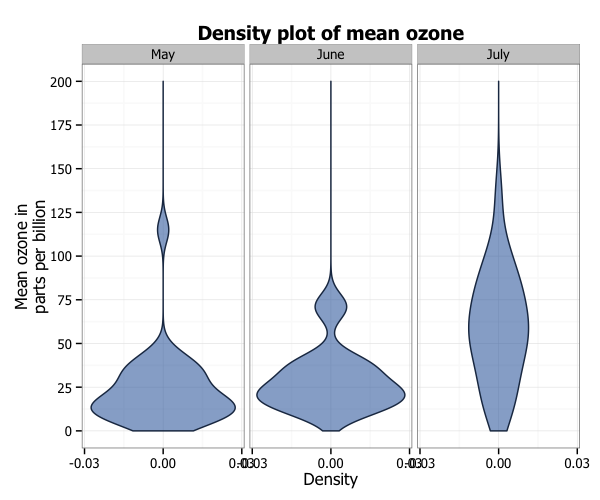In order to plot the three months in the same plot, we add several things. Firstly, in the ggplot function, we add a fill = Month.f argument to aes. Secondly, in order to more clearly see the graph, we add the argument position = "identity" to the geom_density option. This controls the position of the curves respectively. Finally, you can customise the colours of the histograms by adding the scale_fill_brewer to the plot from the RColorBrewer package. This blog post describes the available packages.

library(RColorBrewer)

p8 <- ggplot(airquality_trimmed, aes(x = Ozone, fill = Month.f)) +
geom_density(position="identity", alpha=0.6) +
scale_x_continuous(name = "Mean ozone in\nparts per billion",
breaks = seq(0, 200, 25),
limits=c(0, 200)) +
scale_y_continuous(name = "Density") +
ggtitle("Density plot of mean ozone") +
theme_bw() +
theme(plot.title = element_text(size = 14, family = "Tahoma", face = "bold"),
text = element_text(size = 12, family = "Tahoma")) +
scale_fill_brewer(palette="Accent")
p8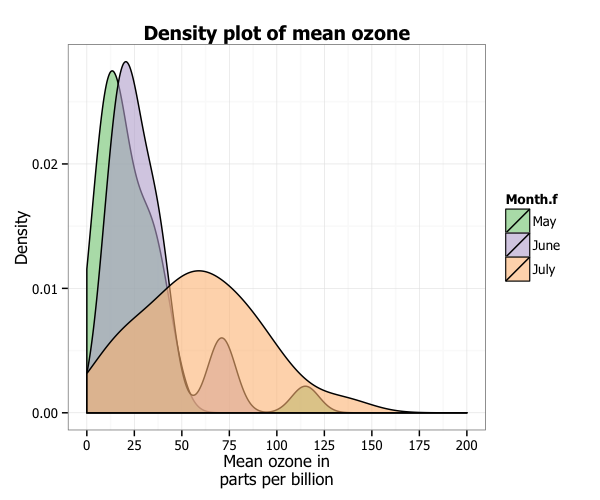These densities are a little hard to see. One way we can make it easier to see them is to stack the densities on top of each other. To do so, we swap position = "stack" for position = "identity" in geom_density.

p8 <- ggplot(airquality_trimmed, aes(x = Ozone, fill = Month.f)) +
geom_density(position = "stack", alpha = 0.6) +
scale_x_continuous(name = "Mean ozone in\nparts per billion",
breaks = seq(0, 200, 25),
limits=c(0, 200)) +
scale_y_continuous(name = "Density") +
ggtitle("Density plot of mean ozone") +
theme_bw() +
theme(plot.title = element_text(size = 14, family = "Tahoma", face = "bold"),
text = element_text(size = 12, family = "Tahoma")) +
scale_fill_brewer(palette="Accent")
p8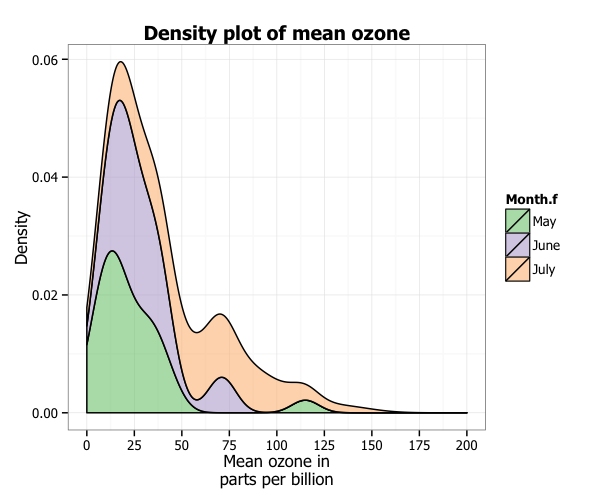Another way to make it a little easier to see the densities by dropping out the fill. To do this need a few changes. We need to swap the option fill = Month.f in ggplot for colour = Month.f. We add the fill = NA to geom_density, and we’ve also added size = 1 to make it easier to see the lines. Finally, we change the scale_fill_brewer() option for scale_colour_brewer().

p8 <- ggplot(airquality_trimmed, aes(x = Ozone, colour = Month.f)) +
geom_density(position="identity", fill = NA, size = 1) +
scale_x_continuous(name = "Mean ozone in\nparts per billion",
breaks = seq(0, 200, 25),
limits=c(0, 200)) +
scale_y_continuous(name = "Density") +
ggtitle("Density plot of mean ozone") +
theme_bw() +
theme(plot.title = element_text(size = 14, family = "Tahoma", face = "bold"),
text = element_text(size = 12, family = "Tahoma")) +
scale_colour_brewer(palette="Accent")
p8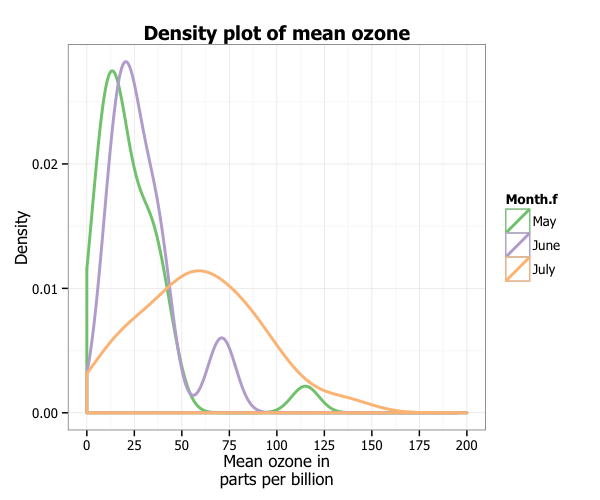### Formatting the legend

Finally, we can format the legend. Firstly, we can change the position by adding the legend.position = "bottom" argument to the theme option, which moves the legend under the plot. Secondly, we can fix the title by adding the labs(fill="Month") option to the plot.

p8 <- ggplot(airquality_trimmed, aes(x = Ozone, colour = Month.f)) +
geom_density(position="identity", fill = NA, size = 1) +
scale_x_continuous(name = "Mean ozone in\nparts per billion",
breaks = seq(0, 200, 25),
limits=c(0, 200)) +
scale_y_continuous(name = "Density") +
ggtitle("Density plot of mean ozone") +
theme_bw() +
theme(plot.title = element_text(size = 14, family = "Tahoma", face = "bold"),
text = element_text(size = 12, family = "Tahoma"),
legend.position = "bottom") +
scale_colour_brewer(palette="Accent") +
labs(colour = "Month")
p8